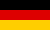# Bri's worldelectronics, programming and more

deutsch# Control theory: control loop PID tuning with step response

For a stable control loop the control parameters have to be set that no oscillations will occur. There are several methods to estimate the parameters for a stable control loop. In this application I use the step response method.

## Control loop

First you have to define the control loop. This is done by selecting the control element types in the table below. The control elements time and gain parameters can be changed in the columns next to the control element type. The Start button is used to draw the control loop step response.

## Control path parameters

Number Control element Parameter K Parameter T Parameter D Transfer function
1
2
3
4

## Control path step response

The step response is used to estimate the PID controller parameters. For this purpose the inflection tangent of the step response is calculated and drawn. The intersection points with the diagram axes are used to calculate the parameters Ks, Tu and Tg. The parameter Ks is calculated from the step response amplitude: $$K_s = \frac{\Delta y}{\Delta u}$$

The control path parameters Ks, Tu and Tg are used to calculate the PID controller parameters Kp, Ki und Kd. The most common calculation rules are these from Ziegler/Nichols and Chien/Hrones/Reswick.

### Calculation rules according to Ziegler/Nichols

Controller Kp Ki Kd
P $$\frac{T_g}{K_s \cdot T_u}$$
PI $$0.9 \cdot \frac{T_g}{K_s \cdot T_u}$$ $$0.273 \cdot \frac{T_g}{K_s \cdot {T_u}^2}$$
PID $$1.2 \cdot \frac{T_g}{K_s \cdot T_u}$$ $$0.6 \cdot \frac{T_g}{K_s \cdot {T_u}^2}$$ $$0.6 \cdot \frac{T_g}{K_s}$$

### Calculation rules according to Chien/Hrones/Reswick

Controller Kp Ki Kd
P $$0.3 \cdot \frac{T_g}{K_s \cdot T_u}$$
PI $$0.35 \cdot \frac{T_g}{K_s \cdot T_u}$$ $$\frac{0.292}{K_s \cdot T_u}$$
PID $$0.6 \cdot \frac{T_g}{K_s \cdot T_u}$$ $$\frac{0.6}{K_s \cdot T_u}$$ $$0.3 \cdot \frac{T_g}{K_s}$$

### Step response calculation

 Step height Step time Simulation end time

### Control path parameters

Parameter Description Value
Ks Gain